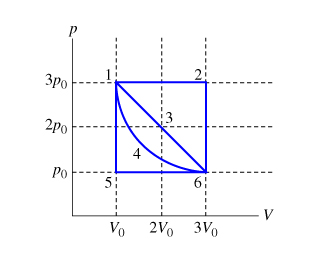# Problem: Calculate the work W done by the gas during process 1 to 3 to 6.Express your answer in terms of p0 and V0.

###### FREE Expert Solution

The work done during process 1-3-6 is given by the area under the curve. We have two shapes that make up the area — A triangle and a rectangle.

Atrangle = (1/2)b•h

Arectangle = l•w

Height, h = 3P0 - P0 = 2P0

Base, b = 3V0 - V0 = 2V0

94% (4 ratings)###### Problem Details

Calculate the work W done by the gas during process 1 to 3 to 6.